# Contrast tests with ART

## Introduction

The aligned-rank transform (ART) allows for non-parametric analyses of variance. But how should we do contrast tests with ART?

Within a single factor, contrasts in ART are straightforward: simply conduct contrasts on the linear model containing the response aligned for that factor.

For interactions, which necessarily involve effects across factors, what types of contrasts are valid may not be immediately obvious. This page explains what types of interaction contrasts are appropriate on aligned-rank-transformed (ART) data, what are not, and why.

## Contents

1. Test Dataset: Description of the test data we will use to compare a linear model against ART
2. Contrast tests of main effects: Demo of conducting contrasts within a single factor (no interaction)
3. Tests of differences in pairwise combinations of levels between factors in interactions: Interaction contrasts that are not valid in ART
4. Tests of differences of differences in interactions: Interaction contrasts that are valid in ART

## Libraries needed for this

library(dplyr)      #data_frame, %>%, filter, summarise, group_by
library(emmeans)    #emmeans, contrast
library(phia)       #testInteractions
library(ARTool)     #art, artlm
library(ggplot2)    #ggplot, stat_..., geom_..., etc

## Test dataset

Let’s generate some test data where we actually know what the effects are. Specifically,

n_per_group = 150
df = data_frame(
X1 = factor(c(rep("A", n_per_group), rep("B", n_per_group))),
X2 = factor(rep(c("C","D","E"), n_per_group*2/3)),
Y = rnorm(n_per_group*2,
(X1 == "B")
+ 2* (X2 == "D")
+ 2 * (X1 == "B" & X2 == "D")
- 2 * (X1 == "A" & X2 == "D")
+ 2 * (X2 == "E"))
)
## Warning: data_frame() is deprecated, use tibble().
## This warning is displayed once per session.

This is normally-distributed error with the same variance at all levels, so we can compare the results of ART to a linear model, which will correctly estimate the effects.

I pre-ran the above code and saved it as InteractionTestData so that the text here is consistent:

data(InteractionTestData)
df = InteractionTestData    #save some typing

The “true” means from the model look like this:

X1 X2 Mean
A C or D 0
A E 2
B C 1
B D 5
B E 3

Which we can see pretty well:

palette = c("#1b9e77", "#d95f02", "#7570b3")
names(palette) = c("C", "D", "E")
ggplot(df, aes(x=X1, y=Y, color=X2)) +
geom_point(pch="-", size=4) +
stat_summary(fun.y=mean, geom="point", size=4) +
stat_summary(fun.y=mean, geom="line", size=1, mapping=aes(group=X2)) +
stat_summary(fun.y=mean, geom="point", size=9, mapping=aes(x=1.5, group=NA), pch="+") +
scale_y_continuous(breaks=seq(-6,10,by=2), minor_breaks=-6:10) +
scale_color_manual(guide=FALSE, values=palette) +
coord_cartesian(ylim=c(-6,10)) +
facet_grid(. ~ X2)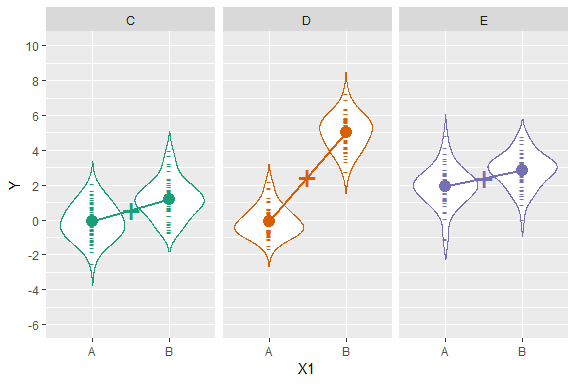And “true” means for each level (averaging over the levels of the other factor):

Level Mean
X1 == A 0.66666
X1 == B 3
X2 == C 0.5
X2 == D 2.5
X2 == E 2.5

Let’s fit a linear model:

m.linear = lm(Y ~ X1*X2, data=df)
anova(m.linear)
## Analysis of Variance Table
##
## Response: Y
##            Df Sum Sq Mean Sq F value    Pr(>F)
## X1          1 445.50  445.50  439.44 < 2.2e-16 ***
## X2          2 236.44  118.22  116.61 < 2.2e-16 ***
## X1:X2       2 270.40  135.20  133.36 < 2.2e-16 ***
## Residuals 294 298.06    1.01
## ---
## Signif. codes:  0 '***' 0.001 '**' 0.01 '*' 0.05 '.' 0.1 ' ' 1

Now with ART:

m.art = art(Y ~ X1*X2, data=df)
anova(m.art)
## Analysis of Variance of Aligned Rank Transformed Data
##
## Table Type: Anova Table (Type III tests)
## Model: No Repeated Measures (lm)
## Response: art(Y)
##
##         Df Df.res F value     Pr(>F)
## 1 X1     1    294  488.33 < 2.22e-16 ***
## 2 X2     2    294  114.35 < 2.22e-16 ***
## 3 X1:X2  2    294  145.65 < 2.22e-16 ***
## ---
## Signif. codes:   0 '***' 0.001 '**' 0.01 '*' 0.05 '.' 0.1 ' ' 1

Both have significance at all levels (expected given the number of samples and the “true” effects) and similar enough F values. The real question is whether/what kind of contrast tests make sense.

### Contrast tests of main effects

For the main effects, let’s look at contrast tests for the linear model:

contrast(emmeans(m.linear, ~ X1), method="pairwise")
##  contrast estimate    SE  df t.ratio p.value
##  A - B       -2.44 0.116 294 -20.963 <.0001
##
## Results are averaged over the levels of: X2
contrast(emmeans(m.linear, ~ X2), method="pairwise")
##  contrast estimate    SE  df t.ratio p.value
##  C - D     -1.9121 0.142 294 -13.428 <.0001
##  C - E     -1.8530 0.142 294 -13.013 <.0001
##  D - E      0.0592 0.142 294   0.415 0.9093
##
## Results are averaged over the levels of: X1
## P value adjustment: tukey method for comparing a family of 3 estimates

These are about right: The “true” effect for A - B is -2.3333, for C - D and C - E is -2, and for D - E is 0 (see table above). From ART:

contrast(emmeans(artlm(m.art, "X1"), ~ X1), method="pairwise")
##  contrast estimate   SE  df t.ratio p.value
##  A - B        -137 6.19 294 -22.098 <.0001
##
## Results are averaged over the levels of: X2
contrast(emmeans(artlm(m.art, "X2"), ~ X2), method="pairwise")
##  contrast estimate   SE  df t.ratio p.value
##  C - D     -123.13 9.28 294 -13.272 <.0001
##  C - E     -119.81 9.28 294 -12.914 <.0001
##  D - E        3.32 9.28 294   0.358 0.9319
##
## Results are averaged over the levels of: X1
## P value adjustment: tukey method for comparing a family of 3 estimates

This is about right (effects in the same direction, the estimates aren’t the same because they are on the scale of ranks and not the data, but the t values are similar to the linear model, as we should hope). Contrast tests of main effects seem to be consistent then.

### Tests of differences in pairwise combinations of levels between factors in interactions

Now let’s look at tests of differences in combinations of levels between factors:

contrast(emmeans(m.linear, ~ X1:X2), method="pairwise")
##  contrast   estimate    SE  df t.ratio p.value
##  A,C - B,C -1.290161 0.201 294  -6.407 <.0001
##  A,C - A,D -0.000506 0.201 294  -0.003 1.0000
##  A,C - B,D -5.113912 0.201 294 -25.395 <.0001
##  A,C - A,E -2.044007 0.201 294 -10.150 <.0001
##  A,C - B,E -2.952089 0.201 294 -14.660 <.0001
##  B,C - A,D  1.289654 0.201 294   6.404 <.0001
##  B,C - B,D -3.823751 0.201 294 -18.988 <.0001
##  B,C - A,E -0.753846 0.201 294  -3.743 0.0030
##  B,C - B,E -1.661928 0.201 294  -8.253 <.0001
##  A,D - B,D -5.113406 0.201 294 -25.392 <.0001
##  A,D - A,E -2.043501 0.201 294 -10.148 <.0001
##  A,D - B,E -2.951583 0.201 294 -14.657 <.0001
##  B,D - A,E  3.069905 0.201 294  15.245 <.0001
##  B,D - B,E  2.161823 0.201 294  10.735 <.0001
##  A,E - B,E -0.908082 0.201 294  -4.509 0.0001
##
## P value adjustment: tukey method for comparing a family of 6 estimates

And for ART:

#DO NOT DO THIS!
contrast(emmeans(artlm(m.art, "X1:X2"), ~ X1:X2), method="pairwise")
##  contrast  estimate   SE  df t.ratio p.value
##  A,C - B,C     76.9 12.4 294   6.202 <.0001
##  A,C - A,D    125.1 12.4 294  10.091 <.0001
##  A,C - B,D    -45.3 12.4 294  -3.650 0.0042
##  A,C - A,E    -12.1 12.4 294  -0.974 0.9258
##  A,C - B,E     87.2 12.4 294   7.030 <.0001
##  B,C - A,D     48.2 12.4 294   3.889 0.0017
##  B,C - B,D   -122.2 12.4 294  -9.853 <.0001
##  B,C - A,E    -89.0 12.4 294  -7.177 <.0001
##  B,C - B,E     10.3 12.4 294   0.828 0.9623
##  A,D - B,D   -170.4 12.4 294 -13.742 <.0001
##  A,D - A,E   -137.2 12.4 294 -11.066 <.0001
##  A,D - B,E    -38.0 12.4 294  -3.062 0.0288
##  B,D - A,E     33.2 12.4 294   2.676 0.0832
##  B,D - B,E    132.4 12.4 294  10.680 <.0001
##  A,E - B,E     99.2 12.4 294   8.004 <.0001
##
## P value adjustment: tukey method for comparing a family of 6 estimates

Very different results.

The linear model tests are easy to interpret: they tell us the expected mean difference between combinations of levels.

The ART results are more difficult to interpret. Take A,C - A,D, which looks like this:

df %>%
filter(X1 == "A", X2 %in% c("C", "D")) %>%
ggplot(aes(x=X1:X2, y=Y, color=X2)) +
geom_point(pch="-", size=4) +
stat_summary(fun.y=mean, geom="point", size=4) +
scale_y_continuous(breaks=seq(-6,10,by=2), minor_breaks=-6:10) +
scale_color_manual(guide=FALSE, values=palette) +
coord_cartesian(ylim=c(-6,10))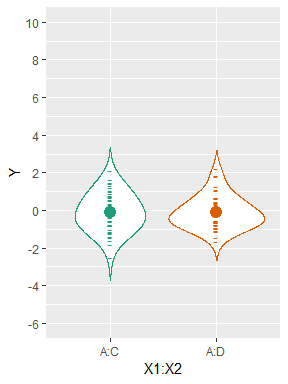The linear model correctly estimates this difference as ~0, which is both the true effect and what we should expect from a visual inspection of the data. Unlike the linear model, the ART model gives us a statistically significant difference between A,C and A,D, which if we interpret in the same way as the linear model is obviously incorrect.

The key here is to understand that ART is reporting differences with the main effects subtracted out. That is, the A,C - A,D effect is something like the difference between this combination of levels if we first subtracted out the effect of C - D. We can see this if we take the ART estimate for C - D in the emmeans for X2 above (-123.13) and the ART estimate for A,C - A,D (125.12) here, we can get approximate an estimate of the difference (-123.13 + 125.12 == 1.99) that is consistent with the expected 0 (given the SE here).

If we first combine the factors before aligning-and-ranking, we can get an estimate of these effects:

#DO NOT DO THIS WITHOUT READING THE NOTE BELOW
df\$X = with(df, X1:X2)
m.art.12 = art(Y ~ X, data=df)
contrast(emmeans(artlm(m.art.12, "X"), ~ X), method="pairwise")
##  contrast  estimate   SE  df t.ratio p.value
##  A:C - A:D     1.96 8.91 294   0.220 0.9999
##  A:C - A:E  -100.96 8.91 294 -11.333 <.0001
##  A:C - B:C   -63.16 8.91 294  -7.090 <.0001
##  A:C - B:D  -205.76 8.91 294 -23.096 <.0001
##  A:C - B:E  -141.84 8.91 294 -15.921 <.0001
##  A:D - A:E  -102.92 8.91 294 -11.553 <.0001
##  A:D - B:C   -65.12 8.91 294  -7.310 <.0001
##  A:D - B:D  -207.72 8.91 294 -23.316 <.0001
##  A:D - B:E  -143.80 8.91 294 -16.141 <.0001
##  A:E - B:C    37.80 8.91 294   4.243 0.0004
##  A:E - B:D  -104.80 8.91 294 -11.764 <.0001
##  A:E - B:E   -40.88 8.91 294  -4.589 0.0001
##  B:C - B:D  -142.60 8.91 294 -16.007 <.0001
##  B:C - B:E   -78.68 8.91 294  -8.832 <.0001
##  B:D - B:E    63.92 8.91 294   7.175 <.0001
##
## P value adjustment: tukey method for comparing a family of 6 estimates

Note that the above table is not in the same order as the previous ones, but does have similar t values to the corresponding effects from the linear model. However, it is not clear this will generalize. In this case there aren’t any other factors except these two, so this is equivalent to just ranking the response before fitting the model, and in the general case it can incorrectly estimate interactions (see issues with using the plain-old rank transform in the ART literature, specifically Higgins’ papers). Thus, I can’t recommended this approach in the general case (or at least, not without more investigation).

### Tests of differences of differences in interactions

While tests of differences of combinations of levels between factors have issues in ART, we can test differences of differences; e.g., for the interaction X1:X2, we might ask, is the difference A - B different when X2 = C compared to when X2 = D? We can test this using the interaction argument to the contrast function in the emmeans package.

Before we test, let’s try to visualize what’s going on in just this interaction:

plot_interaction_for_X2_levels = function(...) {
x2_levels = c(...)
df. = filter(df, X2 %in% x2_levels)
X1_in_X2 = df. %>%
group_by(X1, X2) %>%
summarise(Y = mean(Y)) %>%
print(ggplot(df., aes(x=X1, y=Y, color=X2)) +
geom_point(pch="-", size=4) +
stat_summary(fun.y=mean, geom="point", size=4) +
stat_summary(fun.y=mean, geom="line", size=1, mapping=aes(group=X2), linetype="dashed") +
geom_errorbar(aes(x=2.2, ymin=A, ymax=B, y=NULL),
data=X1_in_X2, width=.19, size=0.8, color="black") +
geom_text(aes(x=2.35, y=(A + B)/2, label=paste("A - B |", X2)),
data=X1_in_X2, hjust=0, size=5, color="black") +
scale_y_continuous(breaks=seq(-6,10,by=2), minor_breaks=-6:10) +
scale_color_manual(guide=FALSE, values=palette[x2_levels]) +
coord_cartesian(xlim=c(0, 3.5), ylim=c(-6,10)) +
facet_grid(. ~ X2))
}
plot_interaction_for_X2_levels("C", "D")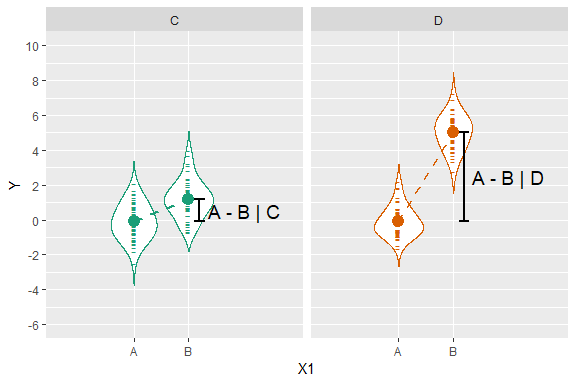The true effect for A - B | C is -1, for A - B | D is -5, and for (A - B | C) - (A - B | D) is (-1) - (-5) = 4. Visually, we’re asking if the two dashed lines in the above plot are parallel. Equivalently, we’re asking if the vertical distance from the mean of A to the mean of B in the left panel (when X2 == C) is the same as the vertical distance between A and B in the right panel (when X2 == D). The true difference between these vertical distances (the “difference of a difference”) is 4, which is also about what we would estimate it to be by looking at the above plot.

We can get the estimate of this “difference of a difference” from the linear model by adding interaction=TRUE to the same call to contrast we made previously:

contrast(emmeans(m.linear, ~ X1:X2), method="pairwise", interaction=TRUE)
##  X1_pairwise X2_pairwise estimate    SE  df t.ratio p.value
##  A - B       C - D          3.823 0.285 294  13.425 <.0001
##  A - B       C - E         -0.382 0.285 294  -1.342 0.1808
##  A - B       D - E         -4.205 0.285 294 -14.766 <.0001

Here we can interpret the row A - B C - D as the difference between (A - B | C) and (A - B | D), which is estimated as 3.82 (close to the true effect of 4, see the plot above).

We can look at a similar plot for the row A - B C - E:

plot_interaction_for_X2_levels("C", "E")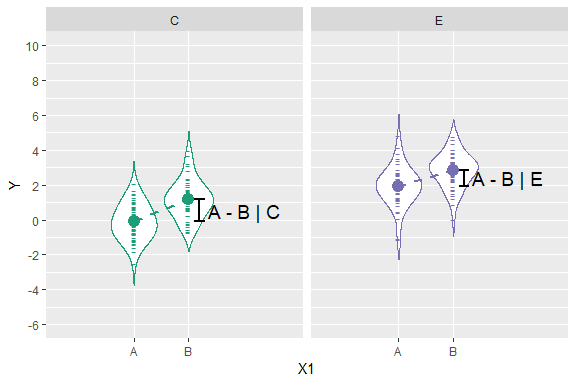Here the true effect for A - B | C is -1, A - B | E is also -1, and (A - B | C) - (A - B | E) is 0. Visually, this sample looks close to the true effects (the height of A - B | C is about the same as A - B | E). From the the row A-B : C-E above we can see that the estimate from the linear model is ~0, as we should hope.

A similar visual analysis finds the estimate for row A - B D - E (~ -4.2) also to be correct (true effect is -4):

plot_interaction_for_X2_levels("D", "E")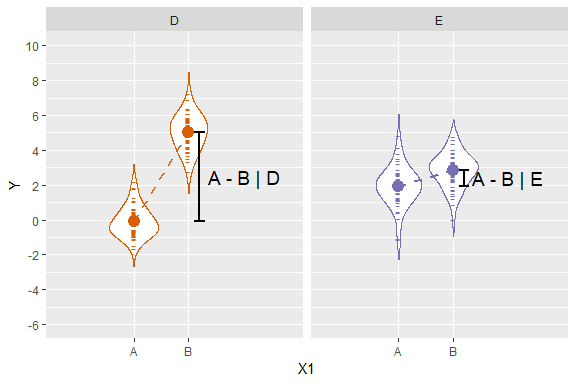Now we look at these differences of differences in art:

contrast(emmeans(artlm(m.art, "X1:X2"), ~ X1:X2), method="pairwise", interaction=TRUE)
##  X1_pairwise X2_pairwise estimate   SE  df t.ratio p.value
##  A - B       C - D          247.3 17.5 294  14.103 <.0001
##  A - B       C - E          -22.3 17.5 294  -1.274 0.2036
##  A - B       D - E         -269.6 17.5 294 -15.377 <.0001

And we see t values consistent with the linear model, and consistent estimates (given the SE). These types of comparisons work under ART because they do not involve coefficients of main effects (see the description of these tests in vignette("phia")), thus are consistent even when we’ve stripped out the main effects.

If you prefer the phia package, the code to run the equivalent tests using the testInteractions function in phia instead of using emmeans is:

testInteractions(artlm(m.art, "X1:X2"), pairwise=c("X1","X2"))
## F Test:
## Signif. codes:  0 '***' 0.001 '**' 0.01 '*' 0.05 '.' 0.1 ' ' 1
While emmeans uses t tests in this case, testInteractions gives the result of equivalent F tests with one numerator degree of freedom (an F test with $$F(1,\nu) = f$$ is equivalent to a two-sided t test with $$t(\nu) = \sqrt{f}$$). I prefer the t test in this case because the t value preserves the direction of the effect (its sign) and is more amenable to calculating interpretable (ish) effect sizes like Cohen’s d. For an example of the latter, see vignette(“art-effect-size”).